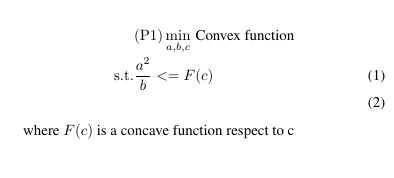# How to deal with this convex problem by cvxI write the constraint by :`F(c)>=a^2 * inv_pos(b)`
Disciplined convex programming error:
Cannot perform the operation: {convex} .* {convex}

I write the constraint by : `F(c)>=a^2 /(b)`
Cannot perform the operation: {convex} ./ {real affine}

How can I solve this problem by CVX?

`a^2/b`, where a and b are both CVX (optimization) variables, is non-convex. Why isn't CVX accepting my model? READ THIS FIRST!

You haven’t shown us enough details of your problem for us to determine whether there may be a reformulation into a form acceptable by CVX. So for now, we will presume that the problem is not convex.

Edit: Ignore my post, except for the link. Go by ncg’s post below.

Given that you seem to enforce that b is positive, `quad_over_lin` should work for you. But do read the FAQ to understand why your approach is wrong. If you understood how to properly use CVX you would not have even attempted it.

Thank you Michael, quad_over_lin work for me.
One more question, how to deal with the a^4/b^2, a and b are both positive variables. I had tried quad_over_lin(square(a),square(b)),
pow_p(a,4).*inv_pos(pow_p(b,2)), they both failed.